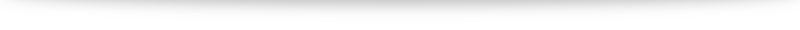# Q3i discussion

Turns out for this question we don't need to use formulas like volume of hemisphere, etc.

Instead, we are given an equation of a curve $x^2 + (y-5)^2 = 25$. We want the volume of the water in the bowl. Able to spot which topic can help us tackle that?